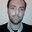Question-and-Answer Resource for the Building Energy Modeling Community
Get started with the Help page

# HVAC output variable that better describes electricity consumptionHi! I'm trying to figure out what are the output variables that better describes HVAC electricity consumption system[Kw] for each hour. I'm using OpenStudio and my single zone model has a Packaged Rooftop Heat Pump HVAC system. It's correct to sum (Air System Total Heating Energy)+(Air System Total Cooling Energy )+(Air System Fan Electric Energy) and then convert [J] to [Kw] in order to obtain electricity consumption? Analysing my *.rdd file I also tried to obtain electricity consumption by using "Facility Total HVAC Electric Demand Power" and the results of this two "methods" are very diferent. What is the best way to obtain the HVAC electricity consumption? Thank you

edit retag close merge delete

Sort by » oldest newest most votedIn Energy plus, you can check mtd file to see what each energy meter is comprised of.

kW is power demand, not energy (kWh) . You should looking for variable that ends with energy not rate.

more

Thank you for your answer Bo_Lin. On InputOutpuReferences we can see that "Facility Total HVAC Electric Demand Power"(HVAC Average [W]) includes all of the electric demand from the HVAC portion of the simulation, which would contain fans, electric coils, pumps, chillers, cooling towers, etc. My simulations are made in a hourly basis. So, as this output variable unit is [W] a can transform it to [Kw]. As I'm working on a hourly basis I can have for each time step my values in [Kwh] (Correct me if I'm wrong).

I'm looking for a output variable that defines HVAC electricity consumption so I can use it in "add_output _variable" measure on OpenStudio and then export it to a spreadsheet using ReadVarESO.